• 傅里叶变换与级数
2022-07-19 16:18:42

参考链接：
DR_CAN老师的原视频

# 0、复习Part4的内容

对于周期为 T T ，即 f ( t ) = f ( t + T ) f\left( t \right) = f\left( {t + T} \right) 的函数，它的傅里叶级数的复数展开形式如下：

f ( t ) = ∑ n = − ∞ ∞ c n e i n ω t f\left( t \right) = \sum\limits_{n = - \infty }^\infty {{c_n}{e^{in\omega t}}} , 其中， c n = 1 T ∫ 0 T f ( t ) e − i n ω t d t {c_n} = \frac{1}{T}\int_0^T {f\left( t \right){e^{ - in\omega t}}dt}

重写一下
f T ( t ) = ∑ n = − ∞ ∞ c n e i n ω 0 t ( 1 ) {f_T}\left( t \right) = \sum\limits_{n = - \infty }^\infty {{c_n}{e^{in{\omega _0}t}}} {\rm{(1)}} ω 0 = 2 π T {\omega _0} = \frac{{2\pi }}{T} 为角频率。

c n = 1 T ∫ 0 T f T ( t ) e − i n ω 0 t d t = 1 T ∫ − T 2 T 2 f T ( t ) e − i n ω 0 t d t ( 2 ) {c_n} = \frac{1}{T}\int_0^T {{f_T}\left( t \right){e^{ - in{\omega _0}t}}dt} = \frac{1}{T}\int_{ - \frac{T}{2}}^{\frac{T}{2}} {{f_T}\left( t \right){e^{ - in{\omega _0}t}}dt} {\rm{(2)}}

在上式中， ∑ n = − ∞ ∞ e i n ω 0 t \sum\limits_{n = - \infty }^\infty {{e^{in{\omega _0}t}}} 可以理解一种规则，而 c n c_n 呢，我们可以理解为它定义了函数， c n = a + b i {c_n} = a + bi 是一个复数。

用图像的表达形式如下我们称上面的图为时域表达，下面的图为频域表达。 工程上一般会取 ∣ c n ∣ \left| {{c_n}} \right| 专门来看幅值。

# 1、由傅里叶级数推导傅里叶变换

对于一个非周期函数，也就说它不重复， T → ∞ T \to \infty

lim ⁡ T → ∞ f T ( t ) = f ( t ) \mathop {\lim }\limits_{T \to \infty } {f_T}\left( t \right) = f\left( t \right)

我们定义，两个频率之间的距离 Δ ω ≜ ( n + 1 ) ω 0 − n ω 0 = ω 0 = 2 π T \Delta \omega \triangleq \left( {n + 1} \right){\omega _0} - n{\omega _0} = {\omega _0} = \frac{{2\pi }}{T}

T ↑ , Δ ω ↓ T \uparrow ,\Delta \omega \downarrow , T → ∞ , Δ ω = 0 T \to \infty ,\Delta \omega = 0

离散的形式→连续的形式，Cn那个图就变成了三维空间的一条连续的曲线啦。 我们横轴的 n ω 0 n{\omega _0} ，也可以变成 ω \omega 啦。我们现在把最开始的（2）式带入到（1）式当中，可以得到
f T ( t ) = ∑ n = − ∞ ∞ 1 T ∫ − T 2 T 2 f T ( t ) e − i n ω 0 t d t e i n ω 0 t {f_T}\left( t \right) = \sum\limits_{n = - \infty }^\infty {\frac{1}{T}\int_{ - \frac{T}{2}}^{\frac{T}{2}} {{f_T}\left( t \right){e^{ - in{\omega _0}t}}dt} {\rm{ }}{e^{in{\omega _0}t}}}

根据 Δ ω \Delta \omega 的定义， Δ ω ≜ 2 π T ⇒ 1 T = 2 π Δ ω \Delta \omega \triangleq \frac{{2\pi }}{T} \Rightarrow \frac{1}{T} = \frac{{2\pi }}{{\Delta \omega }} 。因此上式可进一步表达为:

f T ( t ) = ∑ n = − ∞ ∞ Δ ω 2 π ∫ − T 2 T 2 f T ( t ) e − i n ω 0 t d t e i n ω 0 t {f_T}\left( t \right) = \sum\limits_{n = - \infty }^\infty {\frac{{\Delta \omega }}{{2\pi }}\int_{ - \frac{T}{2}}^{\frac{T}{2}} {{f_T}\left( t \right){e^{ - in{\omega _0}t}}dt} {e^{in{\omega _0}t}}}

此时，我令 T → ∞ T \to \infty

f T ( t ) → f ( t ) {f_T}\left( t \right) \to f\left( t \right)

∫ − T 2 T 2 d t → ∫ − ∞ ∞ d t \int_{ - \frac{T}{2}}^{\frac{T}{2}} {dt} \to \int_{ - \infty }^\infty {dt}

n ω 0 → ω n{\omega _0} \to \omega

∑ n = − ∞ ∞ Δ ω → ∫ − ∞ ∞ d ω \sum\limits_{n = - \infty }^\infty {\Delta \omega } \to \int_{ - \infty }^\infty {d\omega }

因此，上式可进一步化为:

f ( t ) = 1 2 π ∫ − ∞ ∞ ∫ − ∞ ∞ f ( t ) e − i ω t d t e i ω t d ω f\left( t \right) = \frac{1}{{2\pi }}\int_{ - \infty }^\infty {\int_{ - \infty }^\infty {f\left( t \right){e^{ - i\omega t}}dt} {e^{i\omega t}}d\omega }

定义

F ( ω ) ≜ ∫ − ∞ ∞ f ( t ) e − i ω t d t F\left( \omega \right) \triangleq \int_{ - \infty }^\infty {f\left( t \right){e^{ - i\omega t}}dt} ， 这就是傅里叶变换

f ( t ) = 1 2 π ∫ − ∞ ∞ F ( ω ) e i ω t d ω f\left( t \right) = \frac{1}{{2\pi }}\int_{ - \infty }^\infty {F\left( \omega \right){e^{i\omega t}}d\omega } ， 这就是傅里叶逆变换

扩展：我们令 s = i ω s = i\omega F ( s ) = ∫ − ∞ ∞ f ( t ) e − s t d t F\left( s \right) = \int_{ - \infty }^\infty {f\left( t \right){e^{ - st}}dt} ，这就是Laplace变换

系列学习链接：欢迎大家点赞、收藏、留言讨论。

傅里叶级数与傅里叶变换_Part0_欧拉公式证明+三角函数和差公式证明

傅里叶级数与傅里叶变换_Part1_三角函数系的正交性

傅里叶级数与傅里叶变换_Part2_周期为2Π的函数展开为傅里叶级数

傅里叶级数与傅里叶变换_Part3_周期为2L的函数展开为傅里叶级数

傅里叶级数与傅里叶变换_Part4_傅里叶级数的复数形式

傅里叶级数与傅里叶变换_Part5_傅里叶级数推导傅里叶变换

傅里叶级数与傅里叶变换_Part6_离散傅里叶变换推导

傅里叶级数与傅里叶变换_Part7_离散傅里叶变换的性质傅里叶变换 傅里叶级数 数学基础
更多相关内容
• 作 者：韩 昊 ...知乎专栏：时间无关的故事 谨以此文献给大连海事大学的吴楠老师，柳晓鸣老师，王新年老师以及张晶泊老师。 转载的同学请保留上面这句话，谢谢。如果还能保留文章来源就更感激不尽了。 ...

作 者：韩 昊

知 乎：Heinrich

微 博：@花生油工人

知乎专栏：与时间无关的故事

谨以此文献给大连海事大学的吴楠老师，柳晓鸣老师，王新年老师以及张晶泊老师。

转载的同学请保留上面这句话，谢谢。如果还能保留文章来源就更感激不尽了。
https://zhuanlan.zhihu.com/p/19763358

p.s.本文无论是cos还是sin，都统一用“正弦波”（Sine Wave）一词来代表简谐波。

## 一、什么是频域

贯穿时域与频域的方法之一，就是传中说的傅里叶分析。傅里叶分析可分为傅里叶级数（Fourier Serie）和傅里叶变换(Fourier Transformation)，我们从简单的开始谈起。

## 二、傅里叶级数(Fourier Series)的频谱

还是举个栗子并且有图有真相才好理解。

如果我说我能用前面说的正弦曲线波叠加出一个带90度角的矩形波来，你会相信吗？你不会，就像当年的我一样。但是看看下图：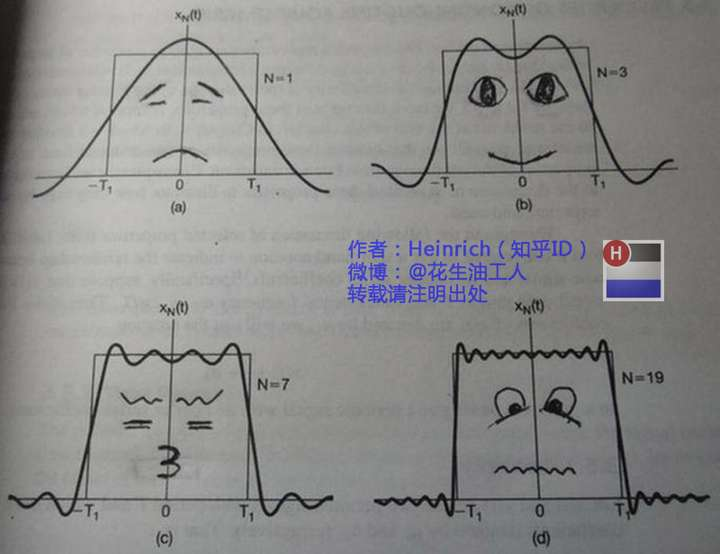第一幅图是一个郁闷的正弦波cos（x）

第二幅图是2个卖萌的正弦波的叠加cos(x)+a.cos(3x)

第三幅图是4个发春的正弦波的叠加

第四幅图是10个便秘的正弦波的叠加

随着正弦波数量逐渐的增长，他们最终会叠加成一个标准的矩形，大家从中体会到了什么道理？

（只要努力，弯的都能掰直！）

随着叠加的递增，所有正弦波中上升的部分逐渐让原本缓慢增加的曲线不断变陡，而所有正弦波中下降的部分又抵消了上升到最高处时继续上升的部分使其变为水平线。一个矩形就这么叠加而成了。但是要多少个正弦波叠加起来才能形成一个标准90度角的矩形波呢？不幸的告诉大家，答案是无穷多个。（上帝：我能让你们猜着我？）

不仅仅是矩形，你能想到的任何波形都是可以如此方法用正弦波叠加起来的。这是没
有接触过傅里叶分析的人在直觉上的第一个难点，但是一旦接受了这样的设定，游戏就开始有意思起来了。

还是上图的正弦波累加成矩形波，我们换一个角度来看看：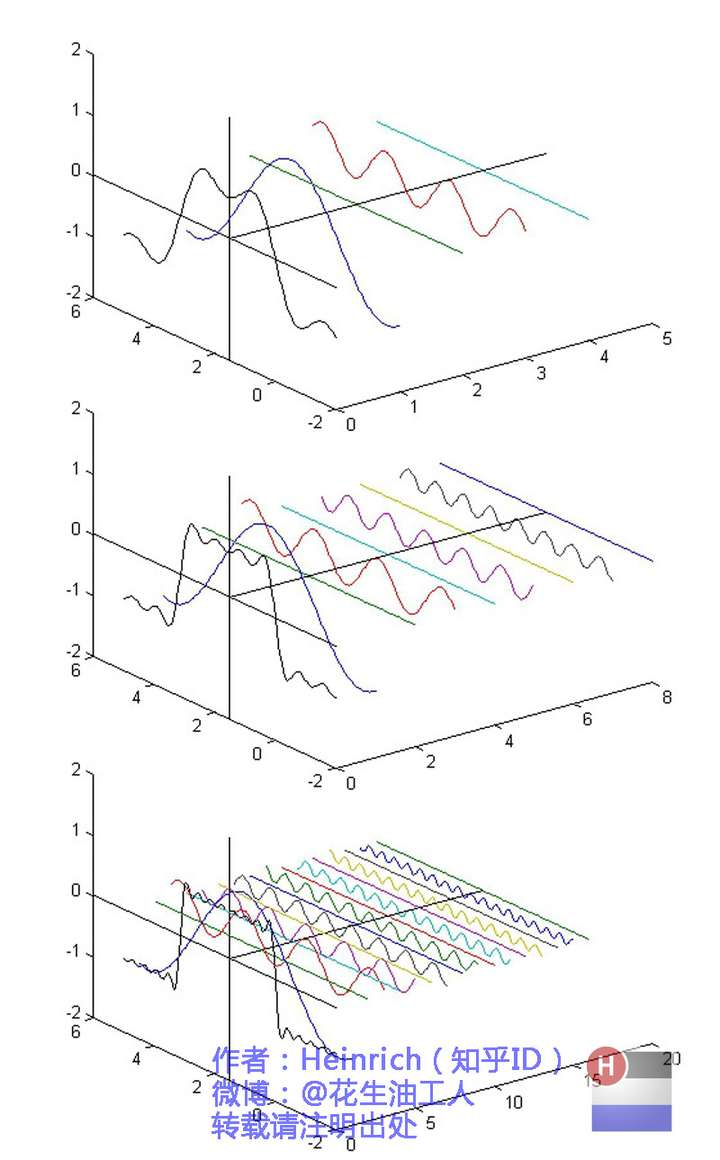在这几幅图中，最前面黑色的线就是所有正弦波叠加而成的总和，也就是越来越接近矩形波的那个图形。而后面依不同颜色排列而成的正弦波就是组合为矩形波的各个分量。这些正弦波按照频率从低到高从前向后排列开来，而每一个波的振幅都是不同的。一定有细心的读者发现了，每两个正弦波之间都还有一条直线，那并不是分割线，而是振幅为0的正弦波！也就是说，为了组成特殊的曲线，有些正弦波成分是不需要的。
这里，不同频率的正弦波我们成为频率分量。
好了，关键的地方来了！！
如果我们把第一个频率最低的频率分量看作“1”，我们就有了构建频域的最基本单元。
对于我们最常见的有理数轴，数字“1”就是有理数轴的基本单元。
时域的基本单元就是“1秒”，如果我们将一个角频率为\omega_{0} 的正弦波cos（\omega_{0} t）看作基础，那么频域的基本单元就是\omega_{0} 。
有了“1”，还要有“0”才能构成世界，那么频域的“0”是什么呢？cos（0t）就是一个周期无限长的正弦波，也就是一条直线！所以在频域，0频率也被称为直流分量，在傅里叶级数的叠加中，它仅仅影响全部波形相对于数轴整体向上或是向下而不改变波的形状。
接下来，让我们回到初中，回忆一下已经死去的八戒，啊不，已经死去的老师是怎么定义正弦波的吧。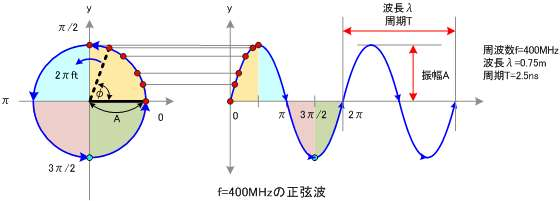正弦波就是一个圆周运动在一条直线上的投影。所以频域的基本单元也可以理解为一个始终在旋转的圆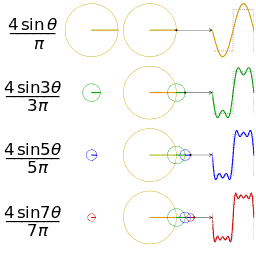介绍完了频域的基本组成单元，我们就可以看一看一个矩形波，在频域里的另一个模样了：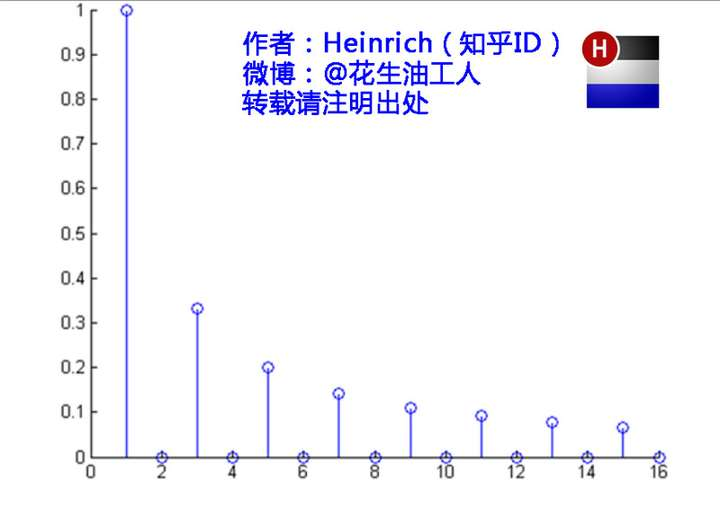这是什么奇怪的东西？
这就是矩形波在频域的样子，是不是完全认不出来了？教科书一般就给到这里然后留给了读者无穷的遐想，以及无穷的吐槽，其实教科书只要补一张图就足够了：频域图像，也就是俗称的频谱，就是——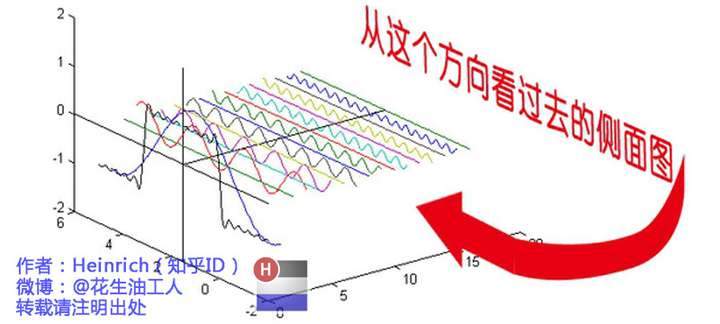再清楚一点：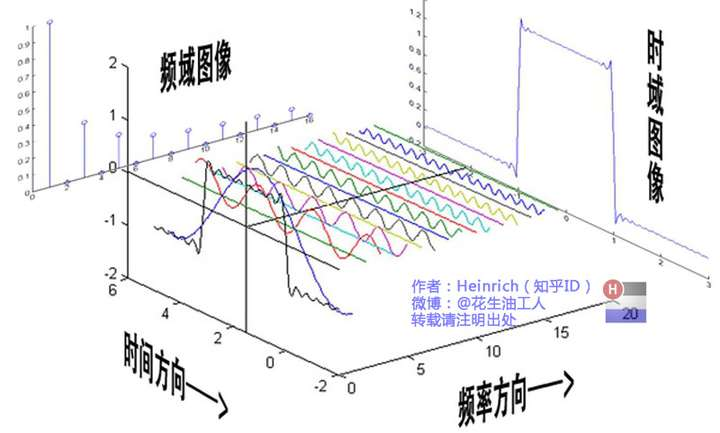可以发现，在频谱中，偶数项的振幅都是0，也就对应了图中的彩色直线。振幅为0的正弦波。

## 三、傅里叶级数（Fourier Series）的相位谱

上一章的关键词是：从侧面看。这一章的关键词是：从下面看。

在这一章最开始，我想先回答很多人的一个问题：傅里叶分析究竟是干什么用的？这段相对比较枯燥，已经知道了的同学可以直接跳到下一个分割线。

先说一个最直接的用途。无论听广播还是看电视，我们一定对一个词不陌生——频道。频道频道，就是频率的通道，不同的频道就是将不同的频率作为一个通道来进行信息传输。下面大家尝试一件事：

先在纸上画一个sin（x），不一定标准，意思差不多就行。不是很难吧。

好，接下去画一个sin（3x）+sin（5x）的图形。

别说标准不标准了，曲线什么时候上升什么时候下降你都不一定画的对吧？

好，画不出来不要紧，我把sin（3x）+sin（5x）的曲线给你，但是前提是你不知道这个曲线的方程式，现在需要你把sin（5x）给我从图里拿出去，看看剩下的是什么。这基本是不可能做到的。

但是在频域呢？则简单的很，无非就是几条竖线而已。

所以很多在时域看似不可能做到的数学操作，在频域相反很容易。这就是需要傅里叶变换的地方。尤其是从某条曲线中去除一些特定的频率成分，这在工程上称为滤波，是信号处理最重要的概念之一，只有在频域才能轻松的做到。

再说一个更重要，但是稍微复杂一点的用途——求解微分方程。（这段有点难度，看不懂的可以直接跳过这段）微分方程的重要性不用我过多介绍了。各行各业都用的到。但是求解微分方程却是一件相当麻烦的事情。因为除了要计算加减乘除，还要计算微分积分。而傅里叶变换则可以让微分和积分在频域中变为乘法和除法，大学数学瞬间变小学算术有没有。

傅里叶分析当然还有其他更重要的用途，我们随着讲随着提。

————————————————————————————————————

下面我们继续说相位谱：

通过时域到频域的变换，我们得到了一个从侧面看的频谱，但是这个频谱并没有包含时域中全部的信息。因为频谱只代表每一个对应的正弦波的振幅是多少，而没有提到相位。基础的正弦波A.sin(wt+θ)中，振幅，频率，相位缺一不可，不同相位决定了波的位置，所以对于频域分析，仅仅有频谱（振幅谱）是不够的，我们还需要一个相位谱。那么这个相位谱在哪呢？我们看下图，这次为了避免图片太混论，我们用7个波叠加的图。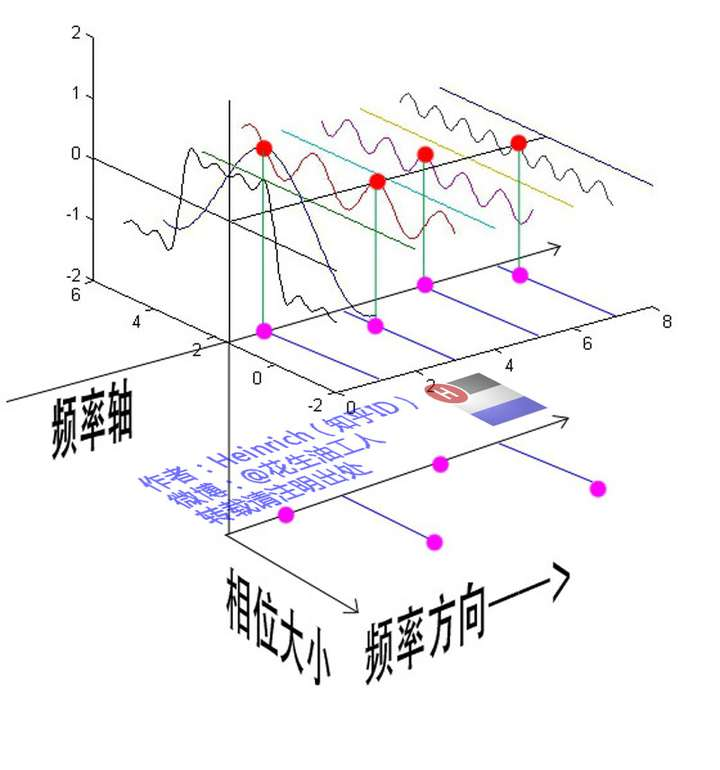鉴于正弦波是周期的，我们需要设定一个用来标记正弦波位置的东西。在图中就是那些小红点。小红点是距离频率轴最近的波峰，而这个波峰所处的位置离频率轴有多远呢？为了看的更清楚，我们将红色的点投影到下平面，投影点我们用粉色点来表示。当然，这些粉色的点只标注了波峰距离频率轴的距离，并不是相位。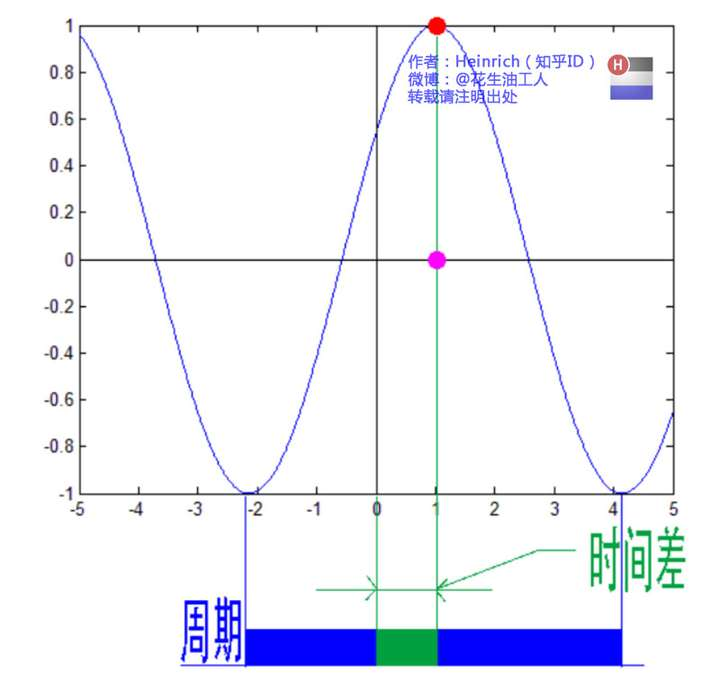这里需要纠正一个概念：时间差并不是相位差。如果将全部周期看作2Pi或者360度的话，相位差则是时间差在一个周期中所占的比例。我们将时间差除周期再乘2Pi，就得到了相位差。
在完整的立体图中，我们将投影得到的时间差依次除以所在频率的周期，就得到了最下面的相位谱。所以，频谱是从侧面看，相位谱是从下面看。下次偷看女生裙底被发现的话，可以告诉她：“对不起，我只是想看看你的相位谱。”

注意到，相位谱中的相位除了0，就是Pi。因为cos（t+Pi）=-cos（t），所以实际上相位为Pi的波只是上下翻转了而已。对于周期方波的傅里叶级数，这样的相位谱已经是很简单的了。另外值得注意的是，由于cos（t+2Pi）=cos（t），所以相位差是周期的，pi和3pi，5pi，7pi都是相同的相位。人为定义相位谱的值域为(-pi，pi]，所以图中的相位差均为Pi。

最后来一张大集合：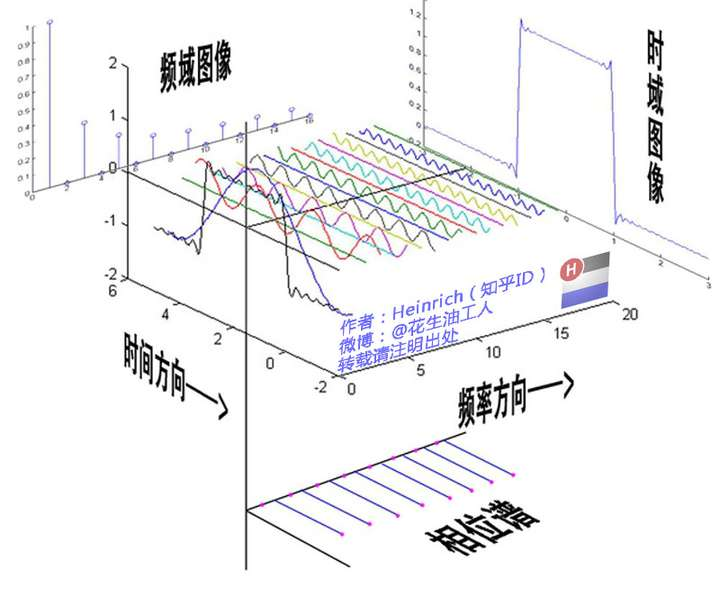## 四、傅里叶变换（Fourier Transformation）

是否有一种数学工具将连续非周期信号变换为周期离散信号呢？抱歉，真没有。

比如傅里叶级数，在时域是一个周期且连续的函数，而在频域是一个非周期离散的函数。这句话比较绕嘴，实在看着费事可以干脆回忆第一章的图片。

而在我们接下去要讲的傅里叶变换，则是将一个时域非周期的连续信号，转换为一个在频域非周期的连续信号。

算了，还是上一张图方便大家理解吧：
比如傅里叶级数，在时域是一个周期且连续的函数，而在频域是一个非周期离散的函数。这句话比较绕嘴，实在看着费事可以干脆回忆第一章的图片。

而在我们接下去要讲的傅里叶变换，则是将一个时域非周期的连续信号，转换为一个在频域非周期的连续信号。

算了，还是上一张图方便大家理解吧：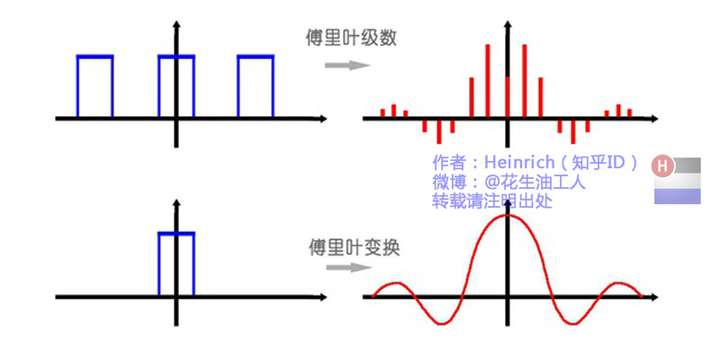或者我们也可以换一个角度理解：傅里叶变换实际上是对一个周期无限大的函数进行傅里叶变换。
所以说，钢琴谱其实并非一个连续的频谱，而是很多在时间上离散的频率，但是这样的一个贴切的比喻真的是很难找出第二个来了。

因此在傅里叶变换在频域上就从离散谱变成了连续谱。那么连续谱是什么样子呢？

你见过大海么？
为了方便大家对比，我们这次从另一个角度来看频谱，还是傅里叶级数中用到最多的那幅图，我们从频率较高的方向看。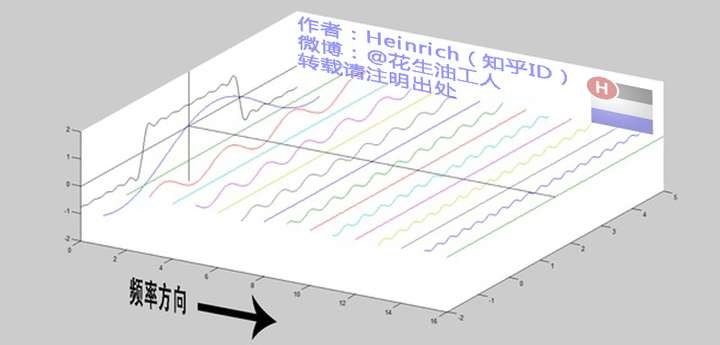以上是离散谱，那么连续谱是什么样子呢？
尽情的发挥你的想象，想象这些离散的正弦波离得越来越近，逐渐变得连续……
直到变得像波涛起伏的大海：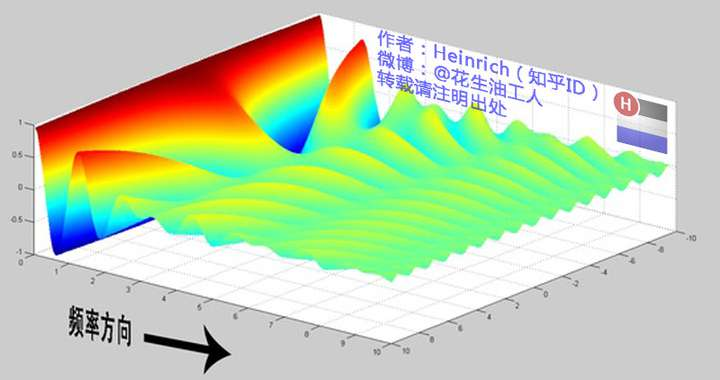很抱歉，为了能让这些波浪更清晰的看到，我没有选用正确的计算参数，而是选择了一些让图片更美观的参数，不然这图看起来就像屎一样了。

不过通过这样两幅图去比较，大家应该可以理解如何从离散谱变成了连续谱的了吧？原来离散谱的叠加，变成了连续谱的累积。所以在计算上也从求和符号变成了积分符号。

不过，这个故事还没有讲完，接下去，我保证让你看到一幅比上图更美丽壮观的图片，但是这里需要介绍到一个数学工具才能然故事继续，这个工具就是——

## 五、宇宙耍帅第一公式：欧拉公式

虚数i这个概念大家在高中就接触过，但那时我们只知道它是-1的平方根，可是它真正的意义是什么呢?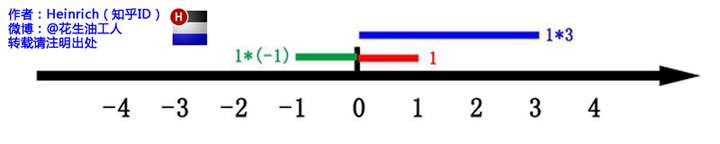这里有一条数轴，在数轴上有一个红色的线段，它的长度是1。当它乘以3的时候，它的长度发生了变化，变成了蓝色的线段，而当它乘以-1的时候，就变成了绿色的线段，或者说线段在数轴上围绕原点旋转了180度。
我们知道乘-1其实就是乘了两次 i使线段旋转了180度，那么乘一次 i 呢——答案很简单——旋转了90度。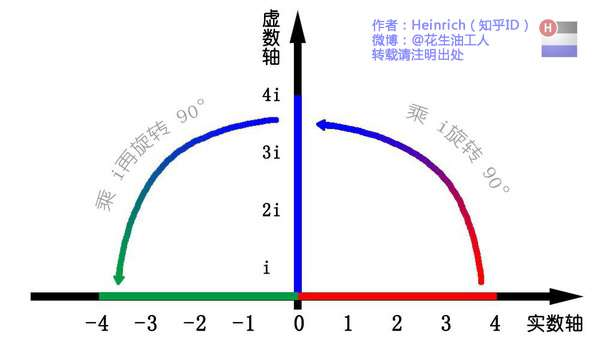同时，我们获得了一个垂直的虚数轴。实数轴与虚数轴共同构成了一个复数的平面，也称复平面。这样我们就了解到，乘虚数i的一个功能——旋转。
现在，就有请宇宙第一耍帅公式欧拉公式隆重登场——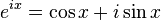这个公式在数学领域的意义要远大于傅里叶分析，但是乘它为宇宙第一耍帅公式是因为它的特殊形式——当x等于Pi的时候。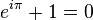经常有理工科的学生为了跟妹子表现自己的学术功底，用这个公式来给妹子解释数学之美：”石榴姐你看，这个公式里既有自然底数e，自然数1和0，虚数i还有圆周率pi，它是这么简洁，这么美丽啊！“但是姑娘们心里往往只有一句话：”臭屌丝……“
这个公式关键的作用，是将正弦波统一成了简单的指数形式。我们来看看图像上的涵义：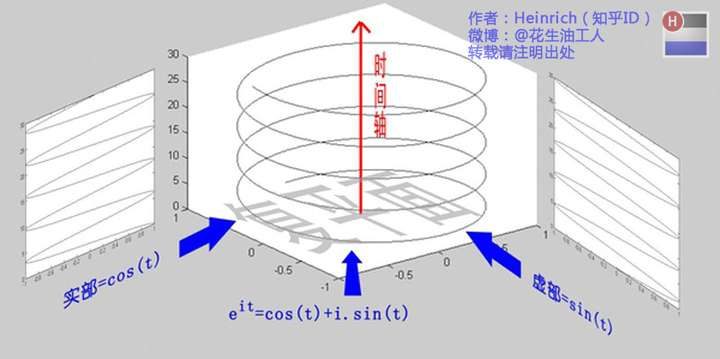欧拉公式所描绘的，是一个随着时间变化，在复平面上做圆周运动的点，随着时间的改变，在时间轴上就成了一条螺旋线。如果只看它的实数部分，也就是螺旋线在左侧的投影，就是一个最基础的余弦函数。而右侧的投影则是一个正弦函数。

## 六、指数形式的傅里叶变换

有了欧拉公式的帮助，我们便知道：正弦波的叠加，也可以理解为螺旋线的叠加在实数空间的投影。
傅里叶变换出来的频谱不仅仅是可见光这样频率范围有限的叠加，而是频率从0到无穷所有频率的组合。
这里，我们可以用两种方法来理解正弦波：
第一种前面已经讲过了，就是螺旋线在实轴的投影。
另一种需要借助欧拉公式的另一种形式去理解：将以上两式相加再除2，得到：这个式子可以怎么理解呢？
我们刚才讲过，e(it)可以理解为一条逆时针旋转的螺旋线，那么e(-it)则可以理解为一条顺时针旋转的螺旋线。而cos(t)则是这两条旋转方向不同的螺旋线叠加的一半，因为这两条螺旋线的虚数部分相互抵消掉了！
好了，刚才我们已经看到了大海——连续的傅里叶变换频谱，现在想一想，连续的螺旋线会是什么样子：

想象一下再往下翻：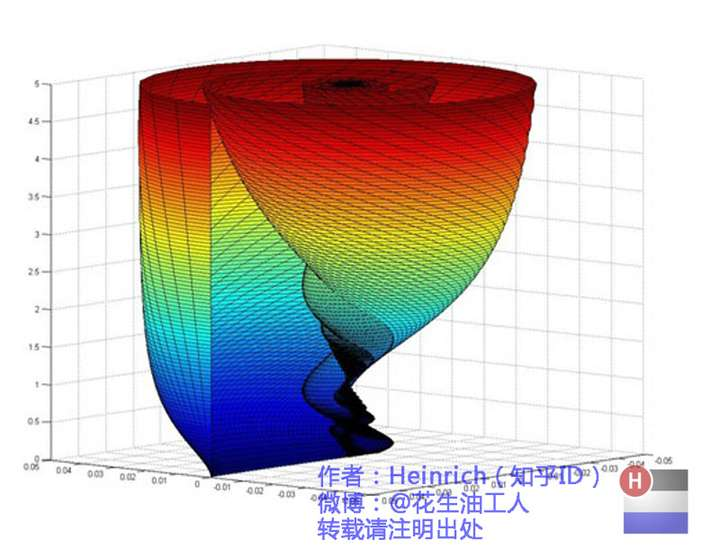是不是很漂亮？
你猜猜，这个图形在时域是什么样子？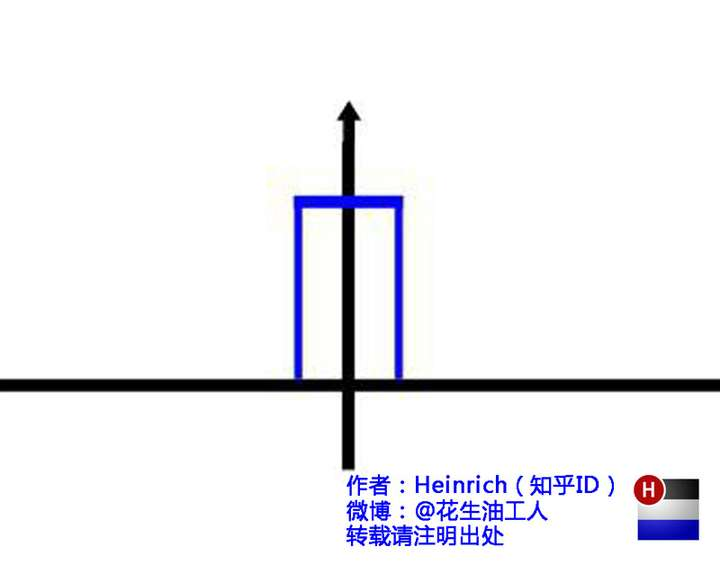哈哈，是不是觉得被狠狠扇了一个耳光。数学就是这么一个把简单的问题搞得很复杂的东西。
顺便说一句，那个像大海螺一样的图，为了方便观看，我仅仅展示了其中正频率的部分，负频率的部分没有显示出来。

如果你认真去看，海螺图上的每一条螺旋线都是可以清楚的看到的，每一条螺旋线都有着不同的振幅（旋转半径），频率（旋转周期）以及相位。而将所有螺旋线连成平面，就是这幅海螺图了。

好了，讲到这里，相信大家对傅里叶变换以及傅里叶级数都有了一个形象的理解了，我们最后用一张图来总结一下：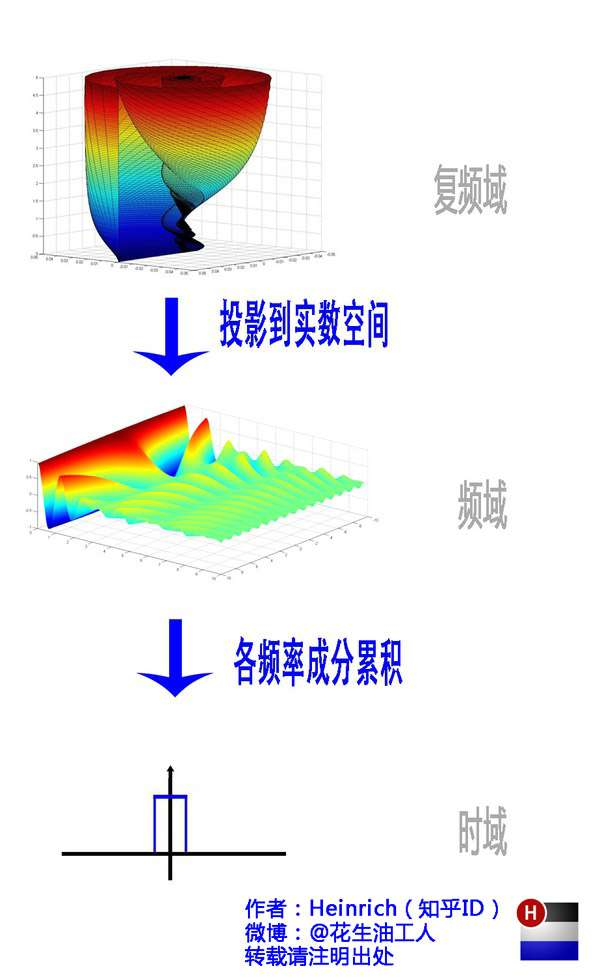到这里，傅里叶的故事就全部讲完了~

展开全文傅里叶变换 傅里叶级数
• 连续时间傅里叶变换(CTFT)离散时间傅里叶变换(DTFT)，以及 傅里叶变换(FT)与傅里叶级数(FS)的关系

## 1. 连续时间傅里叶变换(CTFT)与离散时间傅里叶变换(DTFT)的关系

连续时间傅里叶变换 (C ontinuous T ime F ourier T ransform ) 和 离散时间傅里叶变换 (D iscrete T ime F ourier T ransform) 数学表达形式不同，但本质是一样的。联系两者的桥梁就是 时域冲激采样

时域冲激信号框图：现从的 2 个角度来计算 x ^ ( t ) \hat{x}(t) 的 Fourier Transform：

• 利用“卷积性质”

原信号和冲激串信号的傅里叶变换为

x ( t ) ↔ F T X ( j w ) δ T ( t ) ↔ F T 2 π T ∑ k = − ∞ ∞ δ ( t − k 2 π T ) \begin{aligned} x(t) &\xleftrightarrow{\mathrm{FT}} X(jw)\\ \delta_T(t) &\xleftrightarrow{\mathrm{FT}} \frac{2\pi}{T}\sum_{k=-\infty}^{\infty} \delta(t - k\frac{2\pi}{T}) \end{aligned}

时域相乘等于频域卷积，则冲激采样信号

x ^ ( t ) = x ( t ) δ T ( t ) ↔ F T 1 2 π X ( j w ) ∗ 2 π T ∑ k = − ∞ ∞ δ ( w − 2 π T k ) = 1 T ∑ k = − ∞ ∞ X ( j w − j 2 π T k ) (1) \begin{aligned} \hat{x}(t) &= x(t) \textcolor{blue}{\delta_T(t)}\\ & \xleftrightarrow{\mathrm{FT}} \frac{1}{2\pi} X(jw) * \textcolor{blue}{\frac{2\pi}{T} \sum_{k=-\infty}^{\infty} \delta (w - \frac{2\pi}{T} k)}\\ &= \textcolor{blue}{\frac{1}{T}} \sum_{k=-\infty}^{\infty} X(jw - \textcolor{blue}{j\frac{2\pi}{T}k}) \end{aligned} \tag{1}

根据此式可知， x ^ ( t ) \hat{x}(t) 的频谱是原信号频谱周期延拓（幅度变小为原来的 1 / T 1/T 倍），其频谱如下图所示。

• 利用 F T \mathrm{FT} 的定义

F T [ x ^ ( t ) ] = ∫ − ∞ ∞ x ^ ( t ) e − j w t d t = ∫ − ∞ ∞ ∑ k = − ∞ ∞ x ( k T ) δ ( t − k T ) e − j w t d t = ∑ k = − ∞ ∞ x ( k T ) ∫ − ∞ ∞ δ ( t − k T ) e − j w t d t = ∑ k = − ∞ ∞ x ( k T ) e − j w k T (2) \begin{aligned} \mathrm{FT}[\hat{x}(t)] &= \int_{-\infty}^{\infty} \hat{x}(t) e^{-jwt} dt \\ &= \int_{-\infty}^{\infty} \textcolor{blue}{\sum_{k=-\infty}^{\infty} x(kT)\delta (t-kT)} e^{-jwt} dt\\ &= \textcolor{blue}{\sum_{k=-\infty}^{\infty} x(kT)} \int_{-\infty}^{\infty} \delta(t - kT) e^{-jwt} dt\\ &= \sum_{k=-\infty}^{\infty} x(kT) e^{-jwkT} \tag{2} \end{aligned}

x ( k T ) = x [ k ] ， w T = Ω x(kT) = x[k]，\textcolor{red}{wT=\Omega} ，上式可化为 ∑ k = − ∞ ∞ x [ k ] e − j Ω k \textcolor{red}{\displaystyle\sum_{k=-\infty}^{\infty} x[k] e^{-j\Omega k}} ，这就是序列 x [ n ] x[n] DTFT

其中， T \textcolor{red}T 是采样时间 ( s a m p l e   t i m e ) (sample\ time) w \textcolor{red}w 是模拟频率 ( r a d / s ) \mathrm {(rad/s)} Ω \textcolor{red}\Omega 是数字频率 ( r a d ) \mathrm {(rad)} ，这一步相当于将模拟角频率归一化到数字角频率 [ 0 , 2 π ] [0, 2\pi] ，换了一个 x x 轴而 y y 轴不变，这也解释了“ DTFTCTFT 的频谱为什么如此相像”。
Ω = w T = 2 π w w s ∈ [ 0 , 2 π ] r a d \Omega = wT = 2\pi \frac{w}{w_s} \in [0, 2\pi] \mathrm{rad}由式 ( 1 ) (1) 可以绘制出 x ^ ( t ) \hat{x}(t) 的频谱，由傅里叶变换的唯一性得式 ( 2 ) (2) 的频谱与式 ( 1 ) (1) 是一样的，这也就可以解释 DTFTCTFT 频谱是相似的（横坐标存在一个缩放罢了） ， DTFTCTFT 本质是一致的，都是由同一个东西推导出来的，只不过两者的数学表达形式不同。

DTFT 的意义：

1. 更符合实际情况。我们通常只能得到离散的数据，但是这些离散的数据仍保留原信号的完整信息 / 频谱（当然是在满足一定条件下）；

2. 对模拟角频率进行归一化后，可忽略“采样时间不同”的影响。

例如： 分别以采样频率 f s 1 = 1 H z , f s 2 = 2 H z f_{s1} = 1 \mathrm{Hz}, f_{s2} = 2 \mathrm{Hz} 对同一信号进行采样，得到的序列 x 1 [ n ] , x 2 [ n ] x_1[n], x_2[n] 肯定不同，但它们 DTFT 的频谱是一样的。

3. DTFTCTFT 本质是一致的，只是数学表达形式不同，因此（有些）连续信号的性质可推广到离散序列来。

例如：

(a). 证明 x [ n ] = 1 ↔ F T 2 π ∑ l = − ∞ ∞ δ ( Ω − 2 π l ) \displaystyle x[n] = 1 \xleftrightarrow{\mathrm{FT}} 2\pi \sum_{l=-\infty}^{\infty} \delta(\Omega - 2\pi l)
x [ n ] x[n] 对应的连续时间信号是 x ( t ) = 1 x(t)=1 ，其傅里叶变换为
x ( t ) ↔ F T 2 π δ ( w ) x(t) \xleftrightarrow{\mathrm{FT}} 2\pi \delta(w)
进行时域冲激串采样，采样时间为 T s T_s :
x ^ ( t ) ↔ F T 2 π T s ∑ l = − ∞ ∞ δ ( w − 2 π T s l ) \hat{x}(t) \xleftrightarrow{\mathrm{FT}} \frac{2\pi}{T_s} \sum_{l=-\infty}^{\infty} \delta(\textcolor{red}w - \frac{2\pi}{T_s}l)
w = Ω T s w = \frac{\Omega}{T_s} :
x ^ ( t ) ↔ F T 2 π T s ∑ l = − ∞ ∞ δ ( Ω T s − 2 π T s l ) \hat{x}(t) \xleftrightarrow{\mathrm{FT}} \frac{2\pi}{T_s} \sum_{l=-\infty}^{\infty} \delta(\textcolor{red}{\frac{\Omega}{T_s}} - \frac{2\pi}{T_s}l)
利用冲激信号的展缩特性化简：
x ^ ( t ) ↔ F T 2 π T s ∑ l = − ∞ ∞ δ ( 1 T s ( Ω − 2 π l ) ) = 2 π T s 1 1 T s ∑ l = − ∞ ∞ δ ( Ω − 2 π l ) = 2 π ∑ l = − ∞ ∞ δ ( Ω − 2 π l ) \begin{aligned} \hat{x}(t) &\xleftrightarrow{\mathrm{FT}} \frac{2\pi}{T_s} \sum_{l=-\infty}^{\infty} \delta\left(\textcolor{red}{\frac{1}{T_s}}(\Omega - 2\pi l) \right)\\ &= \frac{2\pi}{T_s} \frac{1}{\textcolor{red}{\frac{1}{T_s}}}\sum_{l=-\infty}^{\infty} \delta\left(\Omega - 2\pi l \right)\\ &= 2\pi \sum_{l=-\infty}^{\infty} \delta\left(\Omega - 2\pi l \right) \end{aligned}
得证！注意 δ ( Ω − 2 π l ) \delta(\Omega-2\pi l) 是一个冲激信号，别和采样序列 δ [ n ] \delta[n] 混淆了。

(b). 单位样本串序列 δ N [ n ] ↔ F T 2 π N ∑ l = − ∞ ∞ δ ( Ω − 2 π l ) \displaystyle \delta_N[n] \xleftrightarrow{\mathrm{FT}} \frac{2\pi}{N} \sum_{l=-\infty}^{\infty} \delta(\Omega - 2\pi l) ，和连续时间的冲激串信号 δ T ( t ) ↔ F T 2 π T ∑ l = − ∞ ∞ δ ( w − 2 π T l ) \displaystyle \delta_T(t) \xleftrightarrow{\mathrm{FT}} \frac{2\pi}{T} \sum_{l=-\infty}^{\infty} \delta(w - \frac{2\pi}{T} l) 有相似的结构，证明方法同上。

## 2. 傅里叶变换(FT)与傅里叶级数(FS)的关系

傅里叶级数 (F ourier S eries cofficients) 的分析对象是周期的信号/序列，它和傅里叶变换之间联系的桥梁是 时域周期延拓 ∗ * 表示卷积运算。以连续时间周期信号 x T ( t ) x_{_T}(t) 为例，有 2 种方法来计算其 FT

• 利用卷积性质

x ( t ) ∗ δ T ( t ) ↔ F T X ( j w ) 2 π T ∑ k = − ∞ ∞ δ ( w − 2 π T k ) = 2 π ∑ k = − ∞ ∞ 1 T X ( j 2 π T k ) δ ( w − 2 π T k ) x(t) * \textcolor{blue}{\delta_{T}(t)} \xleftrightarrow{\mathrm{FT}} X(jw) \textcolor{blue}{\frac{2\pi}{T} \sum_{k=-\infty}^{\infty}\delta(w-\frac{2\pi}{T}k) } = 2\pi \sum_{k=-\infty}^{\infty} \textcolor{red}{\frac{1}{T}X(j\frac{2\pi}{T}k)} \delta(w-\frac{2\pi}{T}k)

• 利用 FS 展开

x T ( t ) = ∑ k = − ∞ ∞ a k e j 2 π T k t ↔ F T ∑ k = − ∞ ∞ a k 2 π δ ( w − 2 π T k ) = 2 π ∑ k = − ∞ ∞ a k δ ( w − 2 π T k ) x_{_T}(t) = \sum_{k=-\infty}^{\infty} a_k e^{j\frac{2\pi}{T}kt} \xleftrightarrow{\mathrm{FT}} \sum_{k=-\infty}^{\infty} a_k \textcolor{blue}{2\pi \delta(w-\frac{2\pi}{T}k) } = 2\pi\sum_{k=-\infty}^{\infty} \textcolor{red}{a_k} \delta(w-\frac{2\pi}{T}k)

对比两式，由 傅里叶变换的唯一性可得

a k = 1 T X ( j 2 π T k ) a_k = \frac{1}{T}X(j\frac{2\pi}{T}k)

对于离散序列，也是类似的推导:

• 利用卷积性质

x [ n ] ∗ δ T [ n ] ↔ F T X ( j Ω ) 2 π N ∑ k = − ∞ ∞ δ ( Ω − 2 π N k ) = 2 π ∑ k = − ∞ ∞ 1 N X ( j 2 π N k ) δ ( Ω − 2 π N k ) x[n] * \textcolor{blue}{\delta_{T}[n]} \xleftrightarrow{\mathrm{FT}} X(j \Omega) \textcolor{blue}{\frac{2\pi}{N} \sum_{k=-\infty}^{\infty}\delta(\Omega-\frac{2\pi}{N}k) } = 2\pi \sum_{k=-\infty}^{\infty} \textcolor{red}{\frac{1}{N}X(j\frac{2\pi}{N}k)} \delta(\Omega-\frac{2\pi}{N}k)

• 利用 FS 展开

x N ( t ) = ∑ k = 0 N − 1 a k e j 2 π T k n ↔ F T ∑ k = 0 N − 1 a k 2 π ∑ l = − ∞ ∞ δ ( w − 2 π N k − 2 π l ) = 2 π ∑ k = 0 N − 1 a k ∑ l = − ∞ ∞ δ ( w − 2 π N k − 2 π l ) = 2 π ∑ k = 0 N − 1 a k ∑ l = − ∞ ∞ δ ( w − 2 π N ( k + N l ) ) = m = k + N l 2 π ∑ m = − ∞ ∞ a k δ ( w − 2 π N m ) \begin{aligned} x_{_N}(t) &= \sum_{k=0}^{N-1} a_k e^{j\frac{2\pi}{T}k n}\\ &\xleftrightarrow{\mathrm{FT}} \sum_{k=0}^{N-1} a_k \textcolor{blue}{2\pi \sum_{l=-\infty}^{\infty} \delta(w-\frac{2\pi}{N}k - 2\pi l) }\\ &= 2\pi\sum_{k=0}^{N-1} \textcolor{red}{a_k} \sum_{l=-\infty}^{\infty} \delta(w-\frac{2\pi}{N}k - 2\pi l)\\ &= 2\pi\sum_{k=0}^{N-1} \textcolor{red}{a_k} \sum_{l=-\infty}^{\infty} \delta\left(w-\frac{2\pi}{N}(k + N l)\right)\\ &\xlongequal{m=k+Nl} 2\pi \sum_{m=-\infty}^{\infty} \textcolor{red}{a_k} \delta\left(w-\frac{2\pi}{N}m \right)\\ \end{aligned}

傅里叶变换的唯一性可得

a k = 1 N X ( j 2 π N k ) a_k = \frac{1}{N}X(j\frac{2\pi}{N}k)

例子：

序列 x [ n ] = R 4 [ n ] = [ 1 , 1 , 1 , 1 ] ( 0 ) x[n]= R_4[n] = [1,1,1,1]_{(0)} ，对其进行周期延拓，周期为 4，得到周期序列 y [ n ] = ( R 4 [ n ] ) 4 y[n]=\left( R_4[n] \right)_4 ，求 y [ n ] y[n] FS

• 根据定义计算： a k = 1 4 ∑ n = 0 3 y [ n ] e − j π 2 k n = 1 4 ⋅ 4 δ [ k ] = δ [ k ] a_k = \displaystyle\frac{1}{4}\sum_{n=0}^{3} y[n] e^{-j\frac{\pi}{2}kn} = \frac{1}{4}\cdot 4\delta[k] = \delta[k]

• 根据 FT 计算：
F T ( x [ n ] ) = ∑ n = − ∞ ∞ x [ n ] e − j w n = ∑ n = 0 3 e − j w n = 1 + e − j w + e − j 2 w + e − j 3 w \begin{aligned} \mathrm{FT}{(x[n])} &= \sum_{n=-\infty}^{\infty} x[n] e^{-jwn}\\ &= \sum_{n=0}^{3} e^{-jwn}\\ &= 1 + e^{-jw} + e^{-j2w} + e^{-j3w} \end{aligned}
a k = 1 N X ( j 2 π N k ) \displaystyle a_k = \frac{1}{N}X(j\frac{2\pi}{N}k)
a 0 = 1 4 ( 1 + e − j ⋅ 0 + e − j 2 ⋅ 0 + e − j 3 ⋅ 0 ) = 1 a 1 = 1 4 ( 1 + e − j ⋅ π 2 + e − j 2 ⋅ π 2 + e − j 3 ⋅ π 2 ) = 0 a 2 = 1 4 ( 1 + e − j ⋅ π + e − j 2 ⋅ π + e − j 3 ⋅ π ) = 0 a 3 = 1 4 ( 1 + e − j ⋅ 3 π 2 + e − j 2 ⋅ 3 π 2 + e − j 3 ⋅ 3 π 2 ) = 0 \begin{aligned} a_0 &= \frac{1}{4} (1 + e^{-j\cdot 0} + e^{-j2\cdot 0} + e^{-j3\cdot 0}) = 1\\ a_1 &= \frac{1}{4} (1 + e^{-j\cdot \frac{\pi}{2}} + e^{-j2\cdot \frac{\pi}{2}} + e^{-j3\cdot \frac{\pi}{2}}) = 0\\ a_2 &= \frac{1}{4} (1 + e^{-j\cdot \pi} + e^{-j2\cdot \pi} + e^{-j3\cdot \pi}) = 0\\ a_3 &= \frac{1}{4} (1 + e^{-j\cdot \frac{3\pi}{2}} + e^{-j2\cdot \frac{3\pi}{2}} + e^{-j3\cdot \frac{3\pi}{2}}) = 0\\ \end{aligned}
结果与根据定义计算的结果相同。

y [ n ] y[n] 实际是一个直流序列，它的周期可以不是 4，如 1 , 2 , 3 , 5 ⋯ 1,2,3,5\cdots ，不同周期的 FS 都可以写为 a k = δ [ k ] a_k=\delta[k] 。 为保证唯一性，傅里叶级数不能单单只看数值，还应该包含信号/序列的基频/周期，才能完整且唯一地表示原信号。
对于连续时间周期信号，FS 有无限项，但是每项代表一个频率分量；对于离散时间周期序列；FS 是有限的、周期的，FS 的个数代表了的原信号的周期。

展开全文• 《难懂的数学》P1：傅里叶变换傅里叶级数与频谱，知识卡片形式的笔记。

## 傅里叶变换、傅里叶级数与频谱

本文系观看B站up主@房东_bili《难懂的数学》系列视频的笔记，整理出来的笔记会以知识卡片的形式呈现，每一P视频对应一篇博文，每一篇博文里会根据内容有若干知识卡片。

原视频如下方：

【难懂的数学】P1-傅里叶变换、傅里叶级数和频谱

# CARD1：从三维运动理解正弦/余弦波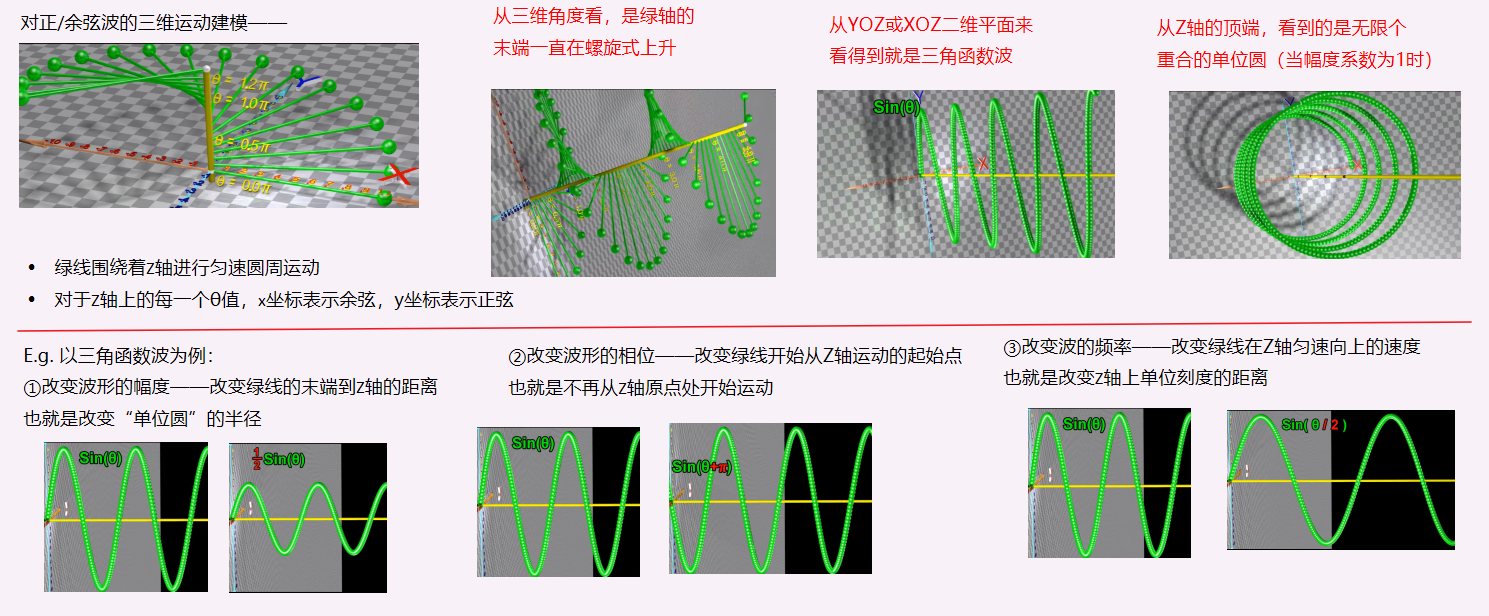# CARD2：以运动的角度观察三角波的运算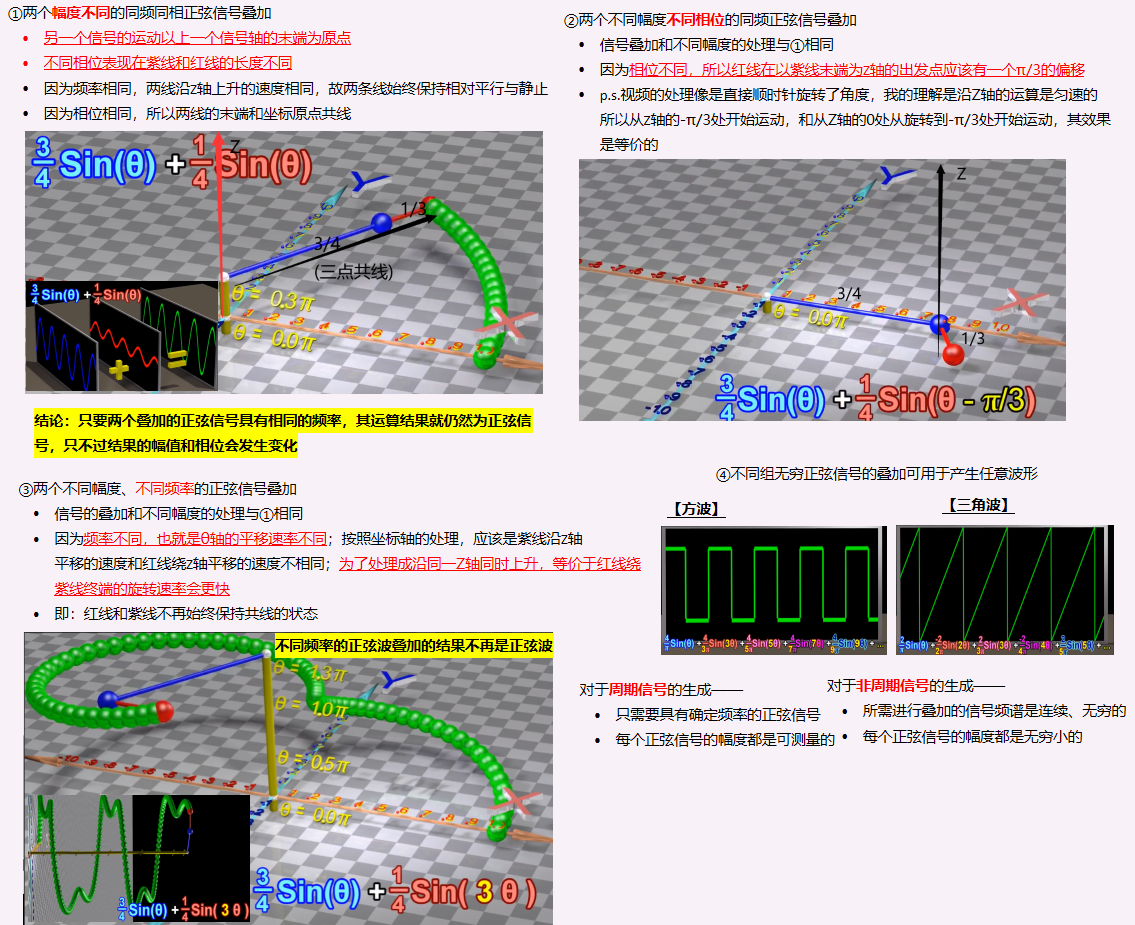# CARD3：以微积分观点理解级数和频谱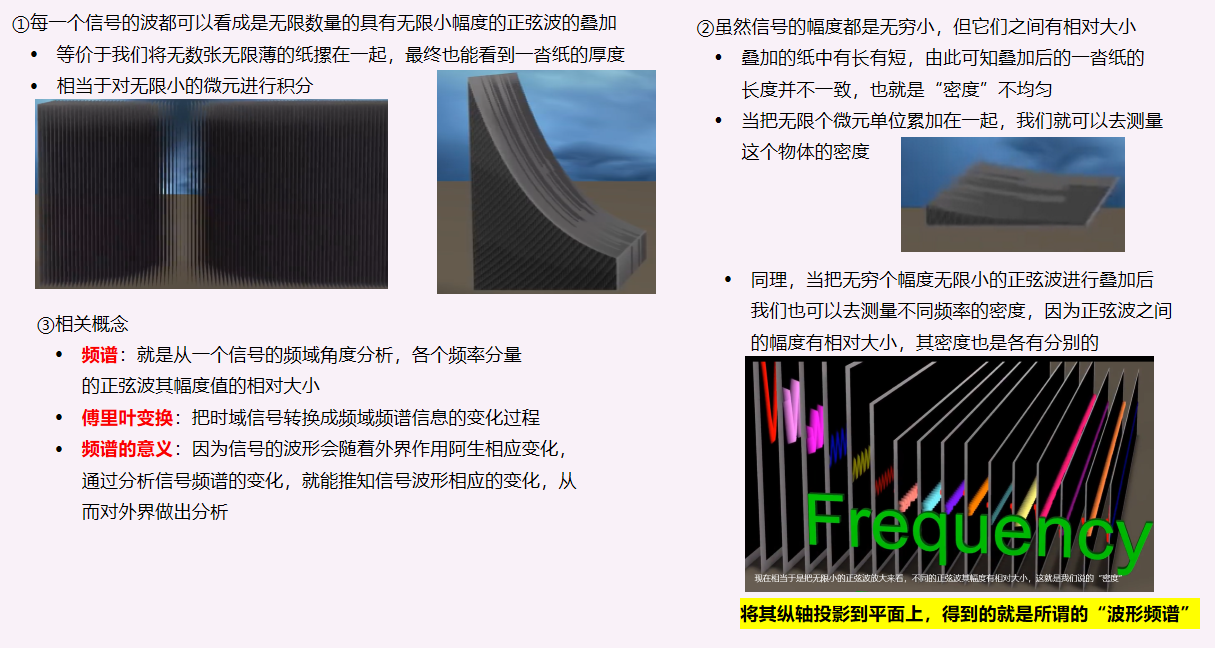展开全文傅里叶变换
• ## 傅里叶级数与傅里叶变换

万次阅读 多人点赞 2019-07-19 17:40:05
傅里叶级数：任何周期函数，只要满足一定条件都可以表示为不同频率的...傅里叶级数与傅里叶变换的关系：周期函数的周期可以趋向无穷大，这样就可以将傅里叶变换看成是傅里叶级数的推广。 一、傅里叶级数 1....
• 基于MATLAB去更形象直观地其学习理解傅里叶级数傅里叶变换，观察频谱的变化，相位的变换，通过例子来明白傅里叶变换与FFT的区别，以及掌握常用的信号处理MATLAB函数
• 以下内容参考《复变函数积分变换》，如果对积分变换有所了解，完全可以跳过忽略 复数的三角表达式如下 Z=r(cosθ+isinθ) Z=r(cos\theta+isin\theta) Z=r(cosθ+isinθ) 欧拉公式如下 eiθ=cosθ+isinθ e^{i\...
• 傅里叶变换傅里叶级数之间的区别联系，详细推导和个人理解。我的CSDN博客中也有这一篇文章。傅里叶变换
• 为了让变换系数不为0，在傅里叶级数的基础上乘了T。 2pi*k/T变成了w。在傅里叶级数中乘了T,逆变换时乘1/T。为了凑出dw(一个角速度增量)。再配一个2pi/2pi,变成了一个2pi/(2piT)，即dw/(2pi)。2pi/T趋近于0，就可看成...
• 快速傅里叶变换的理论应用FFT的简介FFT的理论分析推导FFT的应用 FFT的简介 快速傅里叶变换（英语：Fast Fourier Transform, FFT），是快速计算序列的离散傅里叶变换（DFT）或其逆变换的方法。傅里叶分析将信号从...傅里叶变换 傅里叶级数
• 首先，傅里叶分析是指把一个周期...傅里叶级数 三角函数系的正交性 三角函数系：{1,sinx,cosx,sin2x,cos2x,…,sinnx,cosnx,…}，它由无数个sinnx和cosnx组成，其中n=0,1,2,…。 正交性： ∫−ππsin⁡nxcos⁡mxdx=0，
• 傅里叶变换简介 在数学上，对任意函数 f(x)，可按某一点进行展开，常见的有泰勒展开和傅里叶展开。 泰勒展开为各阶次幂函数的线性组合形式， 本质上自变量未改变，仍为 x。但是傅里叶变换有： 傅里叶展开为三角...傅里叶变换推导 傅里叶级数推导
• 1.傅里叶级数傅里叶变换 笔记来源：fourier series and fourier transform 1.1 正弦波 1.1.1 正弦波相位 改变相位（对应上面第一幅动图中的杆，其初始位置） 1.1.2 正弦波振幅 改变振幅（对应上面第一幅动图...傅里叶变换 傅里叶级数
• 傅里叶级数与傅里叶变换_Part4_傅里叶级数的复数形式 参考链接： DR_CAN老师的原视频 0、复习Part3的内容 参考链接： 傅里叶级数与傅里叶变换_Part3_周期为2L的函数展开为傅里叶级数 对于周期为 T T T，即 f ( t ) =...傅里叶变换 傅里叶级数 数学基础
• 通过傅里叶级数展开，将周期信号分解为一系列“加权”的正弦信号的叠加，对傅里叶级数展开各个频率分量的系数CnC_nCn​，通过将周期信号的周期趋近无穷大，进而得到了非周期信号的傅里叶变换F(jω)F(j\omega)F(jω)...
• > 如果你只会通过公式计算傅里叶级数傅里叶变化，不理解他们的关系 > 通过本文可以让你重新理解傅里叶变换级数
• 离散傅里叶级数，离散傅里叶变换及逆傅里叶变换的实现。
• 傅里叶变换傅里叶级数是有史以来最伟大的数学发现之一。...更不要说学好《信号与系统》、《通信原理》这些专业课程了，所以本文就从不一样的角度来研究它的本质，来揭开傅里叶级数与傅里叶变换的神秘面纱。...数据挖掘 人工智能 dsp开发
• 此文档为个人原创首发，详细介绍了傅里叶变换与N维空间向量正交分解的对应关系，欢迎阅读，如有兴趣可本作者联系，共同学习
• 傅里叶级数仅适用于周期信号，傅里叶变换可以视作傅里叶级数的延伸，可以用于分析非周期信号的频谱特性。事实上，引入冲击函数后，周期信号也可以进行傅里叶变换傅里叶级数：所有周期信号都可以分解为不同频率的...傅里叶变换 傅里叶级数
• 关于傅里叶变换和离散傅里叶级数求和范围的理解 理解： important！！！ 傅里叶的精髓是基函数的正交展开，用n项独立不相关的基频函数可以线性组合出目标函数就够啦！ 基于这一思想，来理解为何有时候积分范围是(−...
• 傅里叶级数与傅里叶变换_Part0_欧拉公式证明+三角函数和差公式证明 1、欧拉公式证明 欧拉公式： e i θ = cos ⁡ θ + i sin ⁡ θ {e^{i\theta }} = \cos \theta + i\sin \theta eiθ=cosθ+isinθ ， 其中， i = ...傅里叶变换 数学基础 傅里叶级数
• 大学专业的通信工程中又一门课程叫做信号系统，本程序就是实现这门课程中的一个知识点，那就是方波和傅里叶级数
• 泰勒级数傅里叶变换、拉普拉斯变换、Z变换简单梳理1、 泰勒级数2、傅里叶系列2.1连续周期信号的傅里叶级数 (FS) 时域连续，频域离散2.2 连续傅里叶变换（FT） 时域连续，频域连续2.3离散周期信号的傅里叶级数 (DFS...
• ## 傅里叶级数及傅里叶变换

万次阅读 多人点赞 2018-06-17 23:59:23
下面通过强度、频率、相位、能量来了解信号（正如通过人的五官去了解人）：正弦信号：周期信号的傅里叶级数：用正弦信号逼近方波：如下图：船的振荡频率为 ，跷跷板的频率为 ，小女孩的玩具的振荡频率为 ，如果......